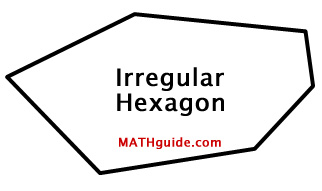What is an Irregular Quadrilateral? To understand what the term 'irregular' means, we need to first look at the term 'regular.' The term regular as it applies to polygons means: all sides are congruent and all internal angles are congruent.     Within the regular pentagon below, the congruent sides have been marked in blue and the congruent angles have been marked in red.Therefore, the term irregular means 'not regular' or that we cannot assume any sides or angles are congruent. The figure below is an example of an irregular hexagon.When polygons are regular, we can make use of congruent central angles. We can divide the polygon into isosceles triangles and then divide those triangles into right triangles, which makes the problem simple for a second year high school student.     When there is no such uniformity, we can only rely on high-powered formulas in trigonometry to aid us. The techniques for approaching irregular quadrilaterals (four-sided polygons) will be discussed below. Area of an Irregular Quadrilateral: SASSS The video directly below will demonstrate how to gain the area of an irregular quadrilateral. For this particular type of quadrilateral, we know the lengths of all four sides and one internal angle.     The steps for finding this area can be done by performing these steps: Divide the figure into two triangles by drawing a diagonal. Calculate the area of the triangle that has the given angle. Calculate the length of the diagonal using the Law of Cosines. Calculate the area of the second triangle using Heron's Formula. Add the two areas of the triangles to determine the area of the quadrilateral.     Watch this video to view all the steps outlined above.ideo: Area of an Irregular Quadrilateral: SASSS Area of an Irregular Quadrilateral: SASAS The video directly below will demonstrate how to gain the area of a different irregular quadrilateral. For this type of quadrilateral, we know the lengths of three sides and two angles enclosed by the known sides.     The steps for finding this area can be done by performing these steps: Divide the figure into two triangles by drawing a diagonal. Calculate the length of the diagonal using the Law of Cosines. Calculate the area of the triangle that has the given (preserved) angle. Calculate the tiny angle (angle 'x') that is a small part of the larger, unpreserved angle using the Law of Sines. Use this angle to find the angle within the second triangle (angle 'y'). Calculate the area of the second triangle. Add the two areas of the triangles to determine the area of the quadrilateral.     Watch this video to view all the steps outlined above.ideo: Area of an Irregular Quadrilateral: SASAS Related Lessons Try these lessons, which are closely related to the lessons above.esson: Law of Cosinesesson: Law of Sinesesson: Area of Regular Polygons